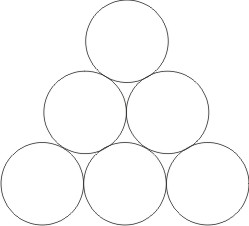# Solving Puzzles Using Linear Programming

## Introduction

Linear programming is an approach to minimising or maximising a linear function of a number of variables subject to a number of constraints.

Specifically, linear progamming seeks to find the values of the variables $$x_1, x_2 …$$ that minimise (or maximise) the function:

$c_1 x_{m+1}+c_2 x_{m+2} + \dots + + c_{n-1} x_{m+n-1}+c_n x_{m+n}$

where $$c_1, c_2 …$$ are constants.  But this minimisation (or maximisation) is subject to a number of specified constraints on the values of the variables:

$\begin{array}xx_1 &=a_{11} x_{m+1}+a_{12} x_{m+2} + \dots + + a_{1,n-1} x_{m+n-1}+a_{1n} x_{m+n} \\ x_2 & =a_{21} x_{m+1}+a_{22} x_{m+2} + \dots + + a_{2,n-1} x_{m+n-1}+a_{2n} x_{m+n} \\ \dots \\ x_m & =a_{n1} x_{m+1}+a_{n2} x_{m+2} + \dots + + a_{n,n-1} x_{m+n-1}+a_{nn} x_{m+n}\end{array}$

and:

$\begin{array} ll_1 \le & x_1 & \le u_1 \\ l_2\le & x_2 & \le u_2 \\ \dots \\ l_{m+n} \le & x_{m+n} & \le u_{m+n}\end{array}$

Here the variables $$x_1 \ldots x_m$$ are referred to colloquially as the row variables, $$x_{m+1} \ldots x_{m+n}$$ as the column variables, $$a_{11} \ldots a_{mn}$$ as the constraint coefficients, $$c_1 \ldots c_n$$ as the objective coefficients, and $$l_1 \ldots l_m$$ and $$u_1\ldots u_m$$ as the lower and upper bounds respectively.

### Puzzle Solving using Linear Programming

To illustrate how linear programming can be used to solve some puzzles, we will use the Love Hearts puzzle. This is repeated here for convenience:

Bobby has made a Valentine card for his girlfriend Chin-We.   He drew rows of identical touching circles in a triangular formation as shown below but with more rows.He then placed a red heart in as many circles as possible without any of these forming equilateral triangles. He continued by placing pink hearts in some of the other circles and white hearts in the remainder.

When he counted the number of hearts of different colours (in no particular order) he obtained three consecutive numbers.

How many red hearts are on the card?

With $$r$$ rows there will be $$r(r+1)/2$$ circles, each of which might have a red heart in it.  So with $$N = r(r+1)/2$$, we first define the variables ($$x_1 \dots x_N$$) whose values will be one if the circle contains a red heart and zero otherwise.  Here the circles and the variables are numbered from $$1 \ldots N$$ starting at the top and proceeding from left to right across successive rows – (1), (2,3),  (4,5,6) …  So our aim is to maximise:

$x_1+x_2+\dots+x_N$
without forming any equilateral triangles.  So for every set of three circles lying on an equilateral triangles, we must find their indexes $$u, v, w$$ and then ensure that:

$x_u+x_v+x_w < 3$

Keeping this value less than three for all sets of three circles prevents the formation of any equilateral triangles.

This set of equations is called a model in Linear Programming jargon.

## Solving with the Gnu Linear Programming Kit (GLPK)

The GNU Linear Programming Kit is a free set of tools for solving linear programming problems.  Its use to solve the puzzle described here is covered here. Here are some of the configurations that the program finds:There is also a solution with 22 red hearts in 11 rows but this takes more than 9 hours to find on my 2GHz i7 machine, which means that the prospect of going further with GLPK is bleak. This set me wondering how ‘a state of the art’ LP solver would achieve.

## Solving with the State of the Art Gurobi SolverLP solvers have important commercial applications in business, finance and industry and  this means that high performance LP solvers require a lot of investment in development and hence justify significant licensing costs. Since I could never justify buying a license for this purely recreational interest, I was not hopeful of being able to go further. But the team at Gurobi were very helpful and offered to provide access to their high performance LP solver for use on this problem. So I am now able to compare the performance of the GLPK and the Gurobi solvers in seeking to extend the number of rows for which we know the maximum possible numbers of red hearts.

With the Gurobi solver I was able to verify that the maximum number of red hearts in 12 rows is 25 (as shown above). Indeed, the performance of the Gurobi solver is good enough to solve for 13 and 14 rows in reasonable times. As can be seen from the comparative timings below, on the 11 row problem Gurobi is faster than GLPK by a factor of 460. This is a very substantial performance advantage and illustrates how the strong commercial drivers for high performance LP solvers have left GLPK far behind.

 rows result GLPK (secs) Gurobi (secs) ratio 9 17 10.8 1.1 10 10 20 137 6.6 21 11 22 32400 70 460 12 25 – 575 – 13 28 – 5420 – 14 31 – 50000 –

My thanks go to the team at Gurobi for providing assistance with this investigation by suggesting how their solver could be tuned for this specific model (not that the default settings were slow!).  The timing figure in red above is an estimate of the time it would take to solve the 14 row problem on my 2GHz i7 machine, based on an actual run on a larger system kindly undertaken by Gurobi.

For those with an interest, here is my model for the problem:

param n, integer, in (1..30);
var a{ i in 1..n, j in 1..i }, integer, >= 0, <= 1;
maximize tri: sum{i in 1..n, j in 1..i } (a[i, j]);
subject to cts {i in 1..n, j in 1..i, k in 1..n-i, m in 0..k-1}:
a[i+m, j] + a[i+k, j+m] + a[i+k-m, j+k-m] <= 2;

data;
param n := 12;
end;